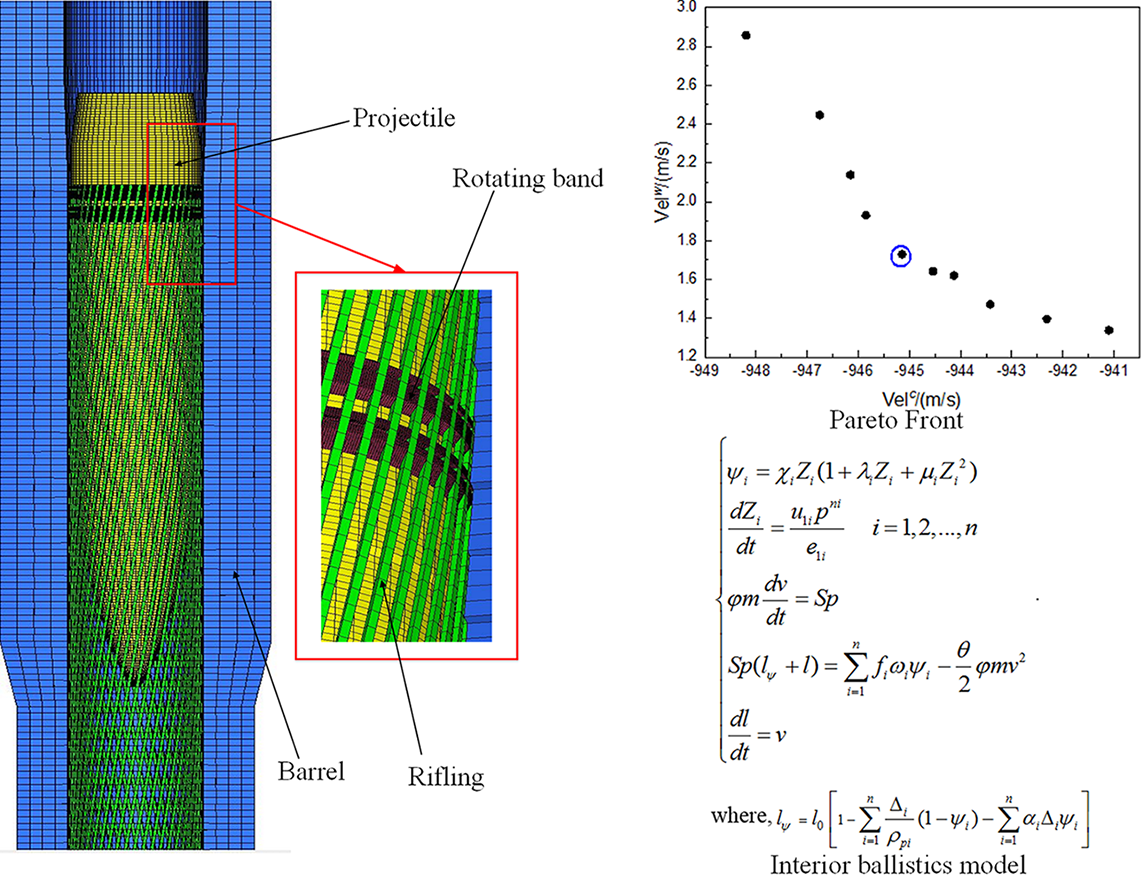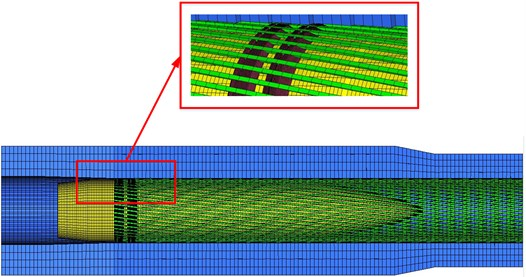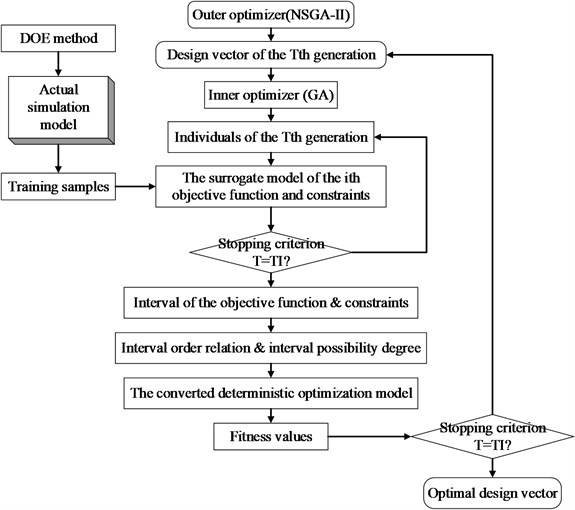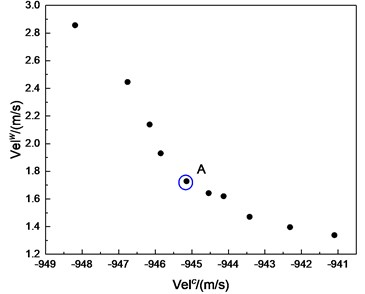Vibroengineering PROCEDIA

Published: 19 October 2019

# Uncertain optimization of in-bore launching performance of artillery based on interval method

Fengjie Xu1
Guolai Yang2
1, 2School of Mechanical Engineering, Nanjing University of Science and Technology, Nanjing, China
Corresponding Author:
Guolai Yang
Views 76

#### Abstract

In order to study the uncertainty optimization of the in-bore launching performance of larger-caliber artillery, the interior ballistic program was compiled and embedded in ABAQUS finite element software for secondary development, and the dynamic model of in-bore launching was built. The structural parameters of bore, the structural parameters of projectile and parameters of launching propellant were considered, and the uncertainty was described by interval number. BP neural network was used to construct a surrogate model for the dynamic model of in-bore launching. The deterministic transformation of uncertain objective function and uncertain constraints was carried out by using interval order model and interval probability model respectively. The interval uncertainty optimization model of the in-bore launching performance artillery was established by taking the interval radius and midpoint of the projectile muzzle velocity as objective functions and the maximum chamber pressure as constraint. Multi-objective genetic algorithm was used to solve the problem, and the optimal solution and reasonable interval of uncertain parameters were obtained.#### Highlights

• The analysis model of artillery in-bore launching performance is established
• The interval method is applied to the uncertain optimization of artillery system
• Finite element model and internal ballistic subroutine

## 1. Introduction

The in-bore launching performance is the key performance of artillery. The motion of projectile in the chamber is affected by many uncertain factors which is the fundamental reason of the difference of ballistic performance. These uncertain factors include: the structural parameters of the gun barrel, the structural parameters of the projectile and parameters of launching propellant.

At present to research in the field of artillery systems rarely involve uncertainty optimization research. Most research focuses on artillery firing dynamics. The research of artillery optimization rarely involves parameter uncertainty. Wang Liqun  used the stochastic robust design theory to optimize the firing density, but the research neglected the stage of projectile motion in the chamber. The design variable optimization results obtained by deterministic optimization of the in-bore launching performance are all deterministic parameter combinations, which are not suitable for solving the uncertain problems in actual production. Therefore, this paper, from the point of view of the integrated design of projectile, gun and propellant, carried out the uncertain optimization of the in-bore launching performance. Firstly, the interior ballistic model and finite model element model were combined to construct the gun in-bore firing dynamics model. Then, based on the interval uncertainty optimization theory, the uncertain optimization model of gun bore firing performance is constructed, and the parameter interval of uncertain design variables is obtained by combining BP neural network surrogate model technology and multi-objective genetic algorithm for optimization solution.

## 2. Artillery in-bore launching model

### 2.1. Finite element mesh model

The gun barrel, projectile and rotating band were modeled discretized. Except for a few wedge-shaped elements at the front of the rotating band, the hexahedral elements with eight nodes were adopted. The deformation of the gun barrel and the projectile is small, so the mesh of this part is large. Influenced by the driving side force, the contact force between the rotating band and rifling is very large, so the mesh size of rotating band should be small. The mesh model is shown in Fig. 1.

Fig. 1Finite element mesh model### 2.2. Interior ballistic model

In the process of projectile motion in the chamber, the energy required for motion is provided by the combustion of the propellant. In order to describe the impact of propellant, an interior ballistic amplitude subroutine (VUAMP) is written to provide the driving force for the projectile. The interior ballistic model adopted the classical interior ballistic model based on thermodynamics, and its mathematical model is shown in Eq. (1):

1

where, ${l}_{\psi }={l}_{0}\left[1-\sum _{i=1}^{n}\frac{{\mathrm{\Delta }}_{i}}{{\rho }_{pi}}\left(1-{\psi }_{i}\right)-\sum _{i=1}^{n}{\alpha }_{i}{\mathrm{\Delta }}_{i}{\psi }_{i}\right]$. $i$ is the number of composite charge, ${\chi }_{i}$, ${\lambda }_{i}$ and ${\mu }_{i}$ are charge form coefficient, $m$ is the mass of projectile, ${\omega }_{i}$ is the mass of charge, $S$ is the equivalent cross-sectional area, ${\phi }_{i}$ is the coefficient of second work, ${l}_{0}$ is the chamber volume-to-bore area ratio, ${l}_{\psi }$ is the free chamber volume-to-bore area ratio, $p$ is the propellant gas pressure, ${\alpha }_{i}$ is the co-volume and $l$ and $v$ are the displacement and velocity of projectile respectively.

In the above formula, the burned thickness $Z$ can be solved by the fourth-order Runge-Kutta method to solve the differential equation. The velocity and displacement of the projectile can be directly obtained by the sensor in ABAQUS, and the combination of the two can solve the chamber pressure. The specific execution process is as follows: the initial chamber pressure is obtained through the initial parameters of the interior ballistic, which pushes the projectile forward at the bottom; the velocity and displacement of the projectile can be read directly by the sensor in ABAQUS as the initial conditions for the next calculation to calculate the next chamber pressure, and so on until the projectile is out of the muzzle. At this point, the artillery in-bore launching model was established. This model can be used to calculate the parameters needed for optimization.

## 3. Uncertain optimization method for artillery in-bore launching performance based on interval optimization

There are generally three uncertain optimization methods: probability model , fuzzy model  and interval model . The interval model is based on interval mathematics and interval programming. The uncertain variables are regarded as the upper and lower intervals of the uniform distribution. It is not necessary to know the probability distribution of the parameters or the fuzzy membership function. Therefore, this paper chooses the interval method to optimize the uncertainty.

The interval uncertain optimization problem can be expressed as:

2

where $\mathbf{X}$ denotes an uncertainty interval vector, which is composed of an $n$-dimensional interval vector ${\mathbf{X}}^{I}$. the superscripts $L$, $R$, $c$ and $w$ represent the lower, upper, center, and radius of the interval, respectively. $f$ and $g$ denote the uncertain objective function and uncertain constraints of the interval optimization, respectively. Due to the transitivity of uncertain factors, objective function and constraints also interval numbers rather than real numbers.

### 3.1. Uncertain design variables

The uncertain design variables considered in this paper include the following three categories (totaling 10). The initial values and ranges of the design variables are listed in Table 1.

a) Projectile parameters: rotating band location ${l}_{d}$, rotating band width $H$, mass eccentricity ${e}_{0}$, CM (center of mass) axial location from shell base ${l}_{R}$, projectile radius $d$.

b) Barrel structure parameters: rifling depth $t$, groove engravings width $b$, chamber volume ${W}_{0}$.

c) Propellant charge parameters: web thickness 2${e}_{1}$, propellant mass $\omega$.

Table 1Initial values and ranges of design variables

 Parameter ${l}_{d}$ (mm) $H$ (mm) ${e}_{0}$ (mm) ${l}_{R}$ (mm) 2${e}_{1}$ (mm) Initial 50.0 27.0 0.0 510.0 2.2 Lower 55.0 30.0 0.0 532.0 2.1 Upper 60.0 33.0 0.5 550.0 2.4 Parameter $d$ (mm) $t$ (mm) $b$ (mm) ${W}_{0}\left(L\right)$ $\omega$ (kg) Initial 76.3 2.325 6.5 26.0 17.0 Lower 74.0 2.325 5.9 25.0 16.4 Upper 77.4 3.1 6.9 27.0 18.0

### 3.2. Treatment of the uncertain objective function

In interval optimization, interval order relations are often used to qualitatively determine whether an interval is better or worse than another interval. In this paper, the interval order relation ${\le }_{cw}$ proposed by Jiang  was adopted to deal with uncertain objective functions. ${A}^{I}{\le }_{cw}{B}^{I}$ means that interval $B$ is better than interval $A$ only when the radius and midpoint of interval $B$ are both smaller than interval $A$. Therefore, the uncertain objective function of Eq. (2) can be transformed into the following multi-objective optimization problem:

3

where, ${f}^{R}\left(\mathbf{X}\right)$ and ${f}^{L}\left(\mathbf{X}\right)$ are upper and lower bounds of the objective function respectively.

### 3.3. Treatment of the uncertain constraints

For the uncertain constraints in Eq. (2), Jiang C.  proposed an improved interval probability degree model to compare the two intervals:

4

where $P\left({A}^{I}\le {B}^{I}\right)$ or $P\left({A}^{I}\ge {B}^{I}\right)$ is the probability of a random variable of interval $A$ being smaller or larger than interval $B$. The uncertain constraints are transformed into deterministic constraints by the interval probability degree model in Eq. (4).

The inequality constraint of type $\le$, such as ${{g}_{i}}^{I}\left(\mathbf{X}\right)\le {{b}_{i}}^{I}$, can be transformed into the following deterministic constraint:

5
$P\left({{g}_{i}}^{I}\left(\mathbf{X}\right)\le {{b}_{i}}^{I}\right)\ge {\lambda }_{i},$

where, ${\lambda }_{i}$ denotes the interval possibility degree value set by the decision maker. Larger value of ${\lambda }_{i}$ indicates stricter constraints.

The inequality constraint of type =, such as ${{g}_{i}}^{I}\left(\mathbf{X}\right)={{b}_{i}}^{I}$, can be transformed into the following two deterministic constraint:

6

Through the above treatment, the interval uncertain optimization problem expressed in Eq. (2) can be converted into the following deterministic optimization problem:

7

where:

From the perspective of launch safety and gun performance, the maximum chamber pressure ${P}_{max}$ and muzzle velocity $Vel$ are selected as constraint and objective function respectively. The maximum chamber pressure and projectile velocity can be obtained by finite element model. Thus, the following deterministic multi-objective optimization model is obtained:

8

The above problem is a two-layer nested optimization problem. The inner optimizer is used to solve the upper and lower bounds of uncertain objective functions and constraints. The outer optimizer is used to search for optimal design variable. Considering that there are two objective functions in Eq. (8), NSGA-II is used to solve the optimal solution. The inner optimizer is the GA. Although the GA has a strong global search capability, its needs too much computation.

In addition, for the artillery in-bore launching model established in this paper, using actual simulation model for nested optimization will bring unacceptable computation cost. In order to solve the contradiction between computational accuracy and computational efficiency, BP neural networks are adopted to replace the initial model. The above method is illustrated in Fig. 2.

Fig. 2Optimization flowchart## 4. Optimization results

A set of non-dominated solutions organized in a Pareto-optimal front is illustrated in Fig. 3. As can be seen from the Fig. 3, as the midpoint of the velocity interval increases, the radius of the interval also increases, which indicates that blindly pursuing high muzzle velocity of the projectile will lead to large velocity fluctuation. Therefore, in order to give consideration to the high muzzle velocity and robustness, point A in the figure $X$ is selected as the final design scheme. The interval of each design variable is obtained and the results are listed in Table 2.

Fig. 3Pareto frontTable 2Intervals of the design variables

 Parameter ${l}_{d}$ (mm) $H$ (mm) ${e}_{0}$ (mm) ${l}_{R}$ (mm) 2${e}_{1}$ (mm) ${X}^{c}$ 55.5998 26.8069 0.1749 529.1584 2.1164 ${X}^{w}$ 0.0823 0.0487 0.0021 5.6190 0.0023 ${X}^{L}$ 55.5175 26.7582 0.1728 523.5394 2.1141 ${X}^{R}$ 55.6821 26.8556 0.177 534.7774 2.1187 Parameter $d$ (mm) $t$ (mm) $b$ (mm) ${W}_{0}\left(L\right)$ $\omega$ (kg) ${X}^{c}$ 76.1279 2.8315 6.2591 26.8069 17.9413 ${X}^{w}$ 0.0014 0.0023 0.0017 0.0487 0.0832 ${X}^{L}$ 76.1265 2.8292 6.2574 26.7582 17.8581 ${X}^{R}$ 76.1293 2.8338 6.2608 26.8556 18.0245

## 5. Conclusions

In this paper, the interval uncertain optimization theory is utilized to research the artillery in-bore launching performance uncertain optimization problem. Reasonable ranges of projectile, gun barrel and propellant charge parameters are obtained. The midpoint of the interval in the optimization result can be regarded as nominal size, and the interval radius can be regarded as tolerance range. It can be used as a reference in the design of artillery weapon system.